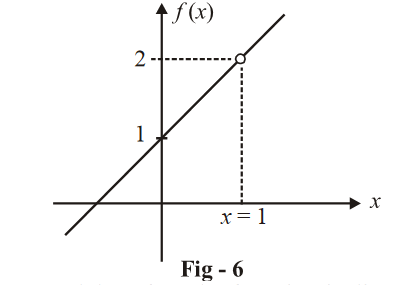# Examples On Continuity Set-4

Go back to  'LCD'

Example – 18

Let f\left( x \right) = \left( {\begin{align}&{\frac{{{a^{2\left[ x \right] + \left\{ x \right\}}} - 1}}{{2\left[ x \right] + \left\{ x \right\}}}} {\qquad x \ne 0}\\&\qquad{\ell n\,a} {\qquad\qquad x = 0}\end{align}} \right). Evaluate the continuity of f(x) at x = 0.

Solution:    The LHL and RHL might differ due to the discontinuous nature of [ x ] and {x}. Lets determine their value:

\begin{align}{\rm{LHL}}\left( {{\rm{at }}x = 0} \right) &= \mathop {\lim }\limits_{h \to 0} f\left( { - h} \right)\\&= \mathop {\lim }\limits_{h \to 0} \frac{{{a^{2\left[ { - h} \right] + \left\{ { - h} \right\}}} - 1}}{{2\left[ { - h} \right] + \left\{ { - h} \right\}}}\end{align}

For h > 0, it is obvious that $$\left[ { - h} \right] = - 1\,{\rm{and }}\left\{ { - h} \right\} = 1 - h.$$

\begin{align}\text{Therefore,} \;{\rm{LHL}} &= \mathop {\lim }\limits_{h \to 0} \frac{{{a^{ - 2 + 1 - h}} - 1}}{{ - 2 + 1 - h}}\\&= \mathop {\lim }\limits_{h \to 0} \frac{{{a^{ - 1 - h}} - 1}}{{ - 1 - h}}\\&= \frac{{{a^{ - 1}} - 1}}{{ - 1}}\\& = {\rm{ }}1 - {a^{ - 1}}\end{align}

Similarly,

\begin{align}{\rm{RHL}} & = \mathop {\lim }\limits_{h \to 0} f\left( h \right)\\ &= \mathop {\lim }\limits_{h \to 0} \frac{{{a^{2\left[ h \right] + \left\{ h \right\}}} - 1}}{{2\left[ h \right] + \left\{ h \right\}}}\\\\ &= \mathop {\lim }\limits_{h \to 0} \frac{{{a^h} - 1}}{h}\\\\& = {\rm{ }}ln\,\,a\end{align}

Since, $${\rm{LHL}} \ne {\rm{RHL}};f\left( x \right)$$  is discontinuous at x = 0.

Note:

(i)   Some authors talk about removable and irremovable discontinuities. Let us discuss what this means:

Consider   \begin{align}f\left( x \right) = \frac{{{x^2} - 1}}{{x - 1}},x \ne 1\end{align}

The LHL and RHL at x = 1 exist and both are equal to 2.There is a hole in the graph at x =1 and therefore the function is discontinuous at x =1 We can, if we want to, fill this hole by redefining the function in the following manner:

f\left( x \right) = \left\{ {\begin{align}&{\frac{{{x^2} - 1}}{{x - 1}}} {\qquad \quad x \ne 1}\\&2 {\qquad\qquad \qquad x = 1}\end{align}} \right\}

The additional definition at x =1 fills the hole and removes the discontinuity. Hence, such a discontinuity would be called a removable discontinuity.

By now, you should have realised that a discontinuity is removable only if the LHL and RHL are equal, since only then we can redefine f (a) to make all the three quantities equal.

If the LHL and RHL are themselves non-equal, no redefinition of f (a) could possibly make the function continuous and hence, such a discontinuity would be called irremovable. For example, f (x) = [x] and f (x) = {x} suffer from irremovable discontinuities at all integers.

(ii)   If f and g are two continuous functions at a point x = a (which is common to their domains), then $$f \pm g$$  and fg will also be continuous at x = a. Furthermore, if $$g\left( a \right) \ne 0,$$ then \begin{align}\,\frac{f}{g}\end{align}  will also be continuous at x = a.

(iii) If g is continuous at x = a and f is continuous at x = g(a), then f(g(x)) will be continuous at    x =a.

(iv) Any polynomial function is continuous for all values of x .

(v)   The functions sin x , cos x and $${{e}^{x}}$$ (or$${{a}^{x}}$$ ) are continuous for all values of x. ln x (or $${{\log }_{a}}x$$) is continuous for all x > 0.

## TRY YOURSELF – III

Q. 1      Find the values of a and f (0) if f (x) is continuous at x = 0, where

\begin{align}&{f\left( x \right) = \frac{{\sin 2x + a\sin x}}{{{x^3}}}} {\quad ,x \ne 0}\end{align}

Q. 2       Find the value of a if f (x) is continuous at x = 0, where

f\left( x \right) = \left\{ {\begin{align}&{\frac{{1 - \cos 4x}}{{{x^2}}}} {\qquad \quad x < 0}\\&a {\qquad \qquad \qquad\quad x = 0}\\&{\frac{{\sqrt x }}{{\sqrt {16 + \sqrt x } - 4}}} {\,\,\,\,\,\,x > 0}\end{align}} \right\}

Q. 3      If the function f (x) defined by

f\left( x \right) = \left\{ {\begin{align}&{\frac{{\log \left( {1 + ax} \right) - \log \left( {1 - bx} \right)}}{x}} {\qquad x \ne 0}\\&\quad\qquad \qquad k \qquad\qquad\qquad\qquad{x = 0}\end{align}} \right\}

is continuous at x = 0, find k.

Q. 4     Let f (xy) = f(x) f(y) for every $$x,y \in \mathbb{R}.$$   If f (x) is continuous at any one point x = a, then prove that  f (x) is continuous for all $$x \in \mathbb{R}\sim\{ 0\}$$ .

Q. 5    Find the values of a and b so that f (x) is continuous at x = 0, where

f\left( x \right) = \left\{ {\begin{align}&\qquad\qquad3 {\quad\quad \quad \quad \quad x = 0}\\&{{{\left( {1 + \frac{{ax + b{x^3}}}{{{x^2}}}} \right)}^{1/x}}} {\,\,\,\,\,\,x \ne 0}\end{align}} \right\}

Q. 6     Discuss the continuity of f (x) = [x] + [–x] at integer points.

Q. 7     Discuss the continuity of f (x) in [0, 2] where

f\left( x \right) = \left\{ {\begin{align}&{\cos \pi x} {\qquad \qquad \quad x \le 1}\\&{\left| {2x - 3} \right|\left[ x \right]} {\qquad \quad x > 1}\end{align}} \right\}

Q. 8    If f\left( x \right) = \left\{ {\begin{align}&{ - 1} {,\qquad \quad x < 0}\\&0 {,\qquad \qquad x = 0}\\&1 {,\qquad \qquad x > 0}\end{align}} \right\}{\rm{and }}\;g\left( x \right) = x\left( {1 - {x^2}} \right), then discuss the continuity of  f (g(x)), and  g (f (x)).